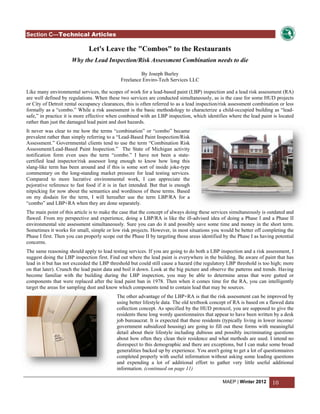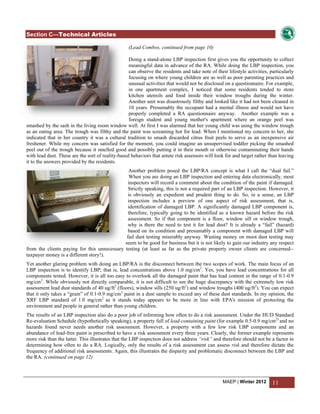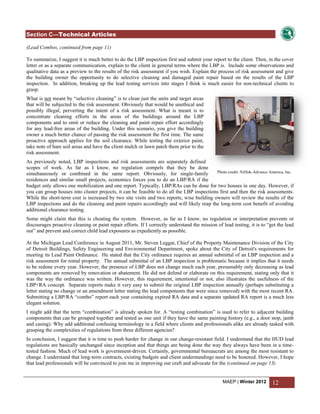Successfully reported this slideshow.

# Why the Lead Paint Testing Combo Should Die×

1 of 4
1 of 4

# Why the Lead Paint Testing Combo Should Die

Outlines the advantages of doing Lead-Based Paint Inspection and Risk Assessment as separate rather than a concurrent scope of services. Advantages to building owners are described as well as an improvement in overall technical quality.

Outlines the advantages of doing Lead-Based Paint Inspection and Risk Assessment as separate rather than a concurrent scope of services. Advantages to building owners are described as well as an improvement in overall technical quality.

## More Related Content

### Why the Lead Paint Testing Combo Should Die

1. 1. 98 ! \$ 7 # %(%, '. 12) ' '3'4+' ') +- /0 0. 0 - )+56) 78 ;=?@B;AHEIJBB A LF EEAAB;? 98 ACDE G B BBK; KMA; ? F E F F NOPRT NVXO QSU WS YSX[S][_QaSUbW_S ccd WSZ ^W` S^R c_SfZOS^W[ [ZRW_ShU RQS Q jQeiW XZ`ZS T_gcNn _RSg[ZkZXZ WR ZRRfSgpqo e [ [_QfSgXS^RgS TR i W Q ZSklRk Z[m o[T_ [ Sk _e RSR [m Q ZSjSX S_S l WrXg[s S gSSg RW_S ZS[VgkRfVg[QROZ _gSR iWRfSuvwTQSg W Xki[k O SVZ_ RtU[ UR jQ S^R W QkS _ X SVXhRRU ZS Q Q Q Z WxR Q d_ Q wSW_W[ZQT[OSW[ShUR_Qg[WiWS gZ ZXZ _RSg[WR ZRRfSgQ _Z_[Q XR W g i gQgSgXVZ XZZRg_ R iS SSWkQ R Sk[T_ y_e RSR [fl[g WSR O Q Q iW X Z ZzQ Q{tUXZWR ZRRfSg_gSlRfSUkXr g ZZgW| Z_`V_kl__rZ zSk QfZX R fls _ _e RSR [ RU Z_ gQQQO Q UWS_S UX QTS VX[ R XZ` O S k k Rih_ TZgS__fQSSi_SjU[fl[kj_ Z cNn_RSg[jU _Sg_RjUW gSXZ T_gRXgk ZS{[ W_ gR W iSg S Q _S g [ [T_ h _U k[_S SSU Sk Z[ _ QZS ^ U Q i WgSgZ xRgSkfZS XZ T_g[ kRU|WR ZUWU[VgU Z rkSk Z[Zk VgZZks }[^WjZ SWg fSUjgSgW zQ _Z_[ Q zQ Q lfS g SS R XZ Q Q U SfR fl[g { W fl{ SZ Q TSZSgZUWgZ RfTOWiW_rgZz Z` Rkn_g[T_[p_e W^X[WgS U[ _ X SSW[ Q cSkNZS Z[}RSg y R Q qRSR [s~QS[ [Z _[Rg[ g VSgSgW dQ _Z_[p_e RRfSg ^WfSgX X g Sk Q R U Sfz fl[g R { S Q qRSR [y Z` Rkn_g}RSg[{ aU bZSQ _Ur[Zg_ RRfSg kNZS Z[ [T_ s S gg i _Z _g cS Q ^O [g_Z_[iW SS VS gSgW zQ Q{}U^ [glS ZRZS Q_g Qf ^[ RR U Sf fls ZS Q S[ gg` i Q W__kXZ _RSgWWR ZRRQ X[ SQr g eQ Uj X[ g_ Sg S Sk [TQy_e RSRW Qr [VU Q [j Q Qr UR i RZrXSgW Z lS ZQ[ Zk_g__RfSRWQ _Rk xe`OS X[`_ SfUR S[ WVk [ iURR Q Qg i[_S QSgT e ffSgW Q gSX[`g[_rfZegTSRW iWXZ gR_rRW_Ss Q [ZO [ U QrRZk[ WS WRVS Q Sk Sg S^R [ dQ ZS g fQS XZ_S S^W[ [Z jQe }[ ZTS_g gS fTWk Q W VWg [_QfSgX Wh Z TWZS U ^ TxWgSWiW[Sg iRiQ ____ i _g[SsNV gZ _SQr SQZ_ SSS Q ZgQk igR[ Zg[Skk gUgR [VU ^ [g_e[ iW[jZQggSRfZgRZkjQk[R Q gSSgW s Rk _ _r Q Q lV U S [_ [ W_SR iUR SfRNZS T Q fOkRZ[iWgSgW }j_ UWZgWVSgSgW cNnpq iWZ [ _k_ Q U Sfh X SSiS R U Sf y Q X zQ Q ZkcNn pqjU[gS ZSk[ RTWgXs fl{ [  S UO W QS SZZSO aU fZ[T_giURZgX_gfZSgSR gZgS[SgiX O k_rgSSRW_S RfVg[QRO_QgZS Zk S _ Q[Q g_ W_SRQ e U ZSUgU QTQ ZjZR Q[ UR S^R_ X SVX R Vkgk [ Z iZ k YQ TWT_SZkSTWSh Q[ ZcNnpq_XSgS_`k_S _S Q k_rZnZS}ZkZnZS} XjSs WffO SRSg [ S_[Sk_r y R_ U XZ^Rk kZ i Q[ UR [ UR } ^ e X S^W[ [ZRgZRRfSgRfVg[QRO bW OV[k _ZkTR_X R^ RfSg ZkfQS _ gSRQggW [_QfSgX_ RSR [ _ X SVXs VS Q Z Q g[ QRlO ZS Q _ [ [O [ U UW Sfs S Z fS bfS_ R_jQe iWR X RfTSQ XjWR TQSgs jSSh[fQg_Z_[ OVjQXl lgWQifTS_rgS Q g g WRQ fZX _ X WQ _e WxRuQ ^W_ RRg g R Q Vk S Sg i Q Xg U fS h VQ S [ nZS}_Rs S OV[TQSXRQSQgU nZS}l gWS_rgQSZSR_Sg_kl gSnZS}RU^[ Tg[_X UR iWgaU[ Q Z WTWO T VgS UR } OZrg UR WZ k[_S OU UR Z Z_r QSg [ i Z [S[s QWR aU RfSWZQ_rRQXZTOgXZ gR_rRW_SsiOVZSr_rgk lgZcNn_RSg[ZkZWR ZRRfSg} S Z SR[[ UVk TX QSkSg S^R} Q W Q[ Q Q QU [T_ [ _e RSR [h [ Q RrSgQ[ gScNn_RSg[iWgY[ Qg SSgSXZ T_gRSSO SS_ gSl__rNSZ W Q T_gUg Z VrRk_r U [T_ _Rs _k V jUW U Sk Z[ _ ^WjUW [ U VX[s jZS i Z[ gZUR Q k XZ __lgZ [gSkkgScNngWRQklgQXR_ VSZU|W mU WrXgW cNngWRQk_gQUr W Sk[gVUR QSSS U USUX VVk gXZR ZZk gSSVZQO USUX RQ _UfQS X Q gZXgWs V gSXZ T_g ZZZkl__kj[cQeZgSlrTVSZkQRWSgSTgWRZkgSks ^[ [ UgZSodW[U U Sk Z[ kg [ QXg Q s Q gU _ _gW [ lS^ U Zg[ [ W[RuZ_r S lfSif_Z j_ gSl__rkW[ gScNn_RSg[ OVfZ l ZX g kgW [ ZSRgZ jSSrgkQ SQ Z XW g U VX[ V_r U [T_ h Q O S lS Q SSf_S WZ Ug W Vg W _ U k Q S fT[[RgZ jSSWTZS ZgWgSXZ T_gl[_ saU[jU[_fS g iWgSpqhOV[_gXr[X Q QSg Ug W SXk iS U Sk Z[ Z [  S S g Q R_ Q U Q Z [SXSg fS _ O gWSgSZSRiWZ XrkRZkeQ _UfT[[Rg[ g[Z[XZ gZfZ l RVR ZrgU WZ Q RfT_ Vg[ [jjU Q QSg SkQ Qg_ SkUg O S QWSs [ aU QUWZ^[ZSQ gScNn pq_gZgSWR ZRRfSgZ l _ W^kl S gS kZgr iU  RUgU _e RSR [[ SfTQS O V_rlgW_SgXkgs SQkglQ [Sgi RlRkQ ZiZ kkg R[ Sg X ROS ZZaU X SgQe QTQ pq_ ZS [ XjS ZZ Si X_[[SgqRRS__kl gSuvwTQQQhQ ZSRTQS g r^ gS QX g QTs TiS OU SQ WgXOV W VTRkQ _SU WRk[RgSSX[ jQk Sg[ZWRgZZTZgU^ lS jWg[l ZkR S_Sg UR Qr WO VR_ [_S UgTSWQ ZS S[ _S O Se Q g xllWZZsgRSTS gZgSSWRk[RmO_ZX X_r_ XjS _fS Q VSVWg}_ Sgk UgUR S_Sg gTX _[ [ Q W[Q y O^ r^WfSgRl__S UV_r ZSr_rg iXQggSSiW j_ fS[[iX QS[ [ VRk|k QR[o W Q[ Q _ V UR QfR g Z_rV X U kg_ZQggSWXSgX _V_rklQRZkTR_X __ [grSg[ SZX lV U_ _ROS [Xk[ V_V [ QRlO [Wf_Z_ VR_ R i [ Q ZQg Q iS gS S[gSWWRk[SZkjUg gQRZSVSs _g[ [ lV UjQg[ UO XZ U_ S_S [ ZfSUk W Rk }[Sk Q kRSTg g_kfQWT_ZkgSSZSST_[hV}[fZSRfSlQk _WRSgQ UR S rZU [ UW W Sg Rlg Z e Q WZ Q r[WX_RlS V l STWSs VZSr_rgrg Xgi VR_[ZWR SSZ_S Zek T O S_[SQ W[gQ[ Q SZQQ Sg [_S g Q fTSS TQSX j_ VSV _iW g[j_QgR_rRfSXZ_rSg[ Q Xgk WTWO g RiX[QfZ_ g V Ze[ Q Sk[ VR_ R U Q U Q ZkST[_rZXgQ Zkg[XSiW g rgS ^W Xg VSV Zkg[X [ Sk[ Q i k_QZ iQg Q ZUW SO _X RiX k_QZ _ gS _ _iW g[   [QfZ_ s     Q  01235 89 67